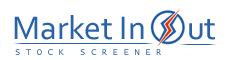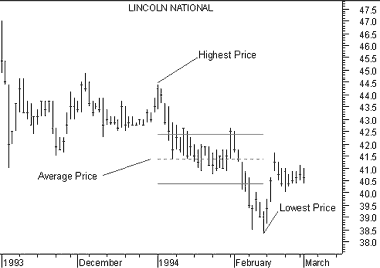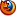Please enable JavaScript to view this page content properly Log In | Sign Up
 Tirone Levels
TIRONE LEVELS

Overview

Tirone Levels are a series of horizontal lines that identify support and resistance levels. They were developed by John Tirone.

Interpretation

Tirone Levels can be drawn using either the Midpoint 1/3-2/3 method or the Mean method. Both methods are intended to help you identify potential support and resistance levels based on the range of prices over a given time period. The interpretation of Tirone Levels is similar to Quadrant Lines.

Example

The following chart shows Midpoint Tirone Levels on Lincoln National.The dotted line shows the average price. The top and bottom lines divide the range between the highest and lowest prices into thirds.

Calculation

Midpoint Method

Midpoint levels are calculated by finding the highest high and the lowest low during the time period being analyzed. The lines are then calculated as follows:

• Top line:

Subtract the lowest low from the highest high, divide this value by three, and then subtract this result from the highest high.

• Center Line:

Subtract the lowest low from the highest high, divide this value by two, and then add this result to the lowest low.

• Bottom Line:

Subtract the lowest low from the highest high, divide this value by three, and then add this result to the lowest low.

Mean Method

Mean levels are displayed as five lines (the spacing between the lines is not necessarily symmetrical). The lines are calculated as follows:

• Extreme High:

Subtract the lowest low from the highest high and add this value to the Adjusted Mean.

• Regular High:

Subtract the lowest low from the value of the Adjusted Mean multiplied by two.

This is the sum of the highest high, the lowest low, and the most recent closing price, divided by three.

• Regular Low:

Subtract the highest high from the value of the Adjusted Mean multiplied by two.

• Extreme Low:

Subtract the lowest low from the highest high and then subtract this value from the Adjusted Mean.

 Best viewed in